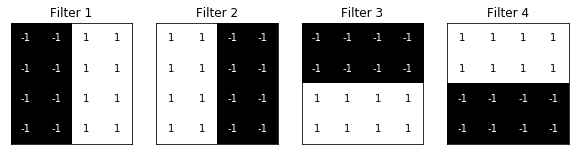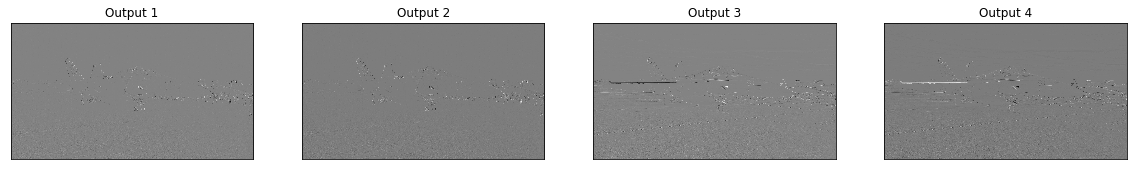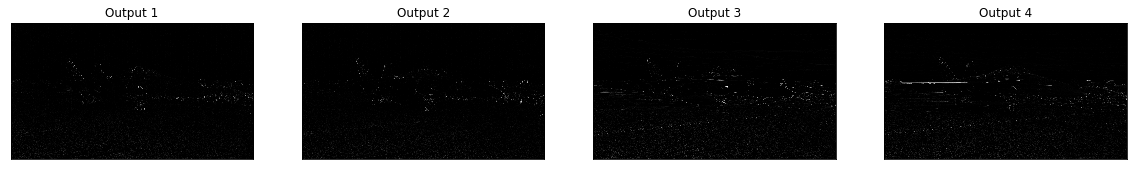# Pytorch Tiny Edge Detection

By Matthew Millar R&D Scientist at ユニファ

### Purpose

This blog will show how to make a custom edge detector using a very small Convolutional Network and Pytorch. This will be a very simple approach to making a fast and accurate Edge detector which is better than Opencv Canny Edge Detector.

### Edge Detection

Edge detection is a basic computer vision technique that allows for the edges or boundaries of an image to be found. This is one of the basic methods for CV that most people do when they start using OpenCV as their Canny edge is fairly good, but will have to be worked over several times to find the optimal parameters per image which cannot be applied to every image that is processed. That is where CNN can help out.

### CNN for edge detection

CNNs are very good at extracting features from an image. These features can then be used to generate edges of the image. The process starts by reading in an image then converting it to a grayscale image. To get good results, building out your own filters would be best, but for ok results using a very basic filter can be used. Then using these filters in CNN as the kernel size will allow for the extraction of features from the image. The activation layer (normally Relu) will give you the black and white extraction that you would expect.

Let's walk through the Code.

The imports you will need

```import cv2
import matplotlib.pyplot as plt
import numpy as np
import torch
import torch.nn as nn
import torch.nn.functional as F
```

Using a photo I took from an American Air Show in Japan we will show how to work with Pytorch and edge detection from scratch.

```img_path = 'data/f18.jpg'
gray = cv2.cvtColor(img, cv2.COLOR_BGR2GRAY)
gray = gray.astype('float32')/255
plt.imshow(gray, cmap='gray')
plt.show()
```

Next we need to define our filters which will do the feature extraction in the CNN

```# Vizulization filters
base_filter = np.array([[-1, -1, 1, 1], [-1, -1, 1, 1], [-1, -1, 1, 1], [-1, -1, 1, 1]])
print("Filers: ", base_filter.shape)
# Defining four different filters,
# all of which are linear combinations of the `filter_vals` defined above

# define four filters
filter_1 = base_filter
filter_2 = -filter_1
filter_3 = filter_1.T
filter_4 = -filter_3
filters = np.array([filter_1, filter_2, filter_3, filter_4])
print('Filter 1: \n', filter_1)
```

Here is what the filters will look like in Numpy

```Filers:  (4, 4)
Filter 1:
[[-1 -1  1  1]
[-1 -1  1  1]
[-1 -1  1  1]
[-1 -1  1  1]]```

That may not look very nice so lets visualize them a bit better.

```# visualize all four filters
fig = plt.figure(figsize=(10, 5))
for i in range(4):
ax = fig.add_subplot(1, 4, i+1, xticks=[], yticks=[])
ax.imshow(filters[i], cmap='gray')
ax.set_title('Filter %s' % str(i+1))
width, height = filters[i].shape
for x in range(width):
for y in range(height):
ax.annotate(str(filters[i][x][y]), xy=(y,x),
horizontalalignment='center',
verticalalignment='center',
color='white' if filters[i][x][y]<0 else 'black')
```FiltersNow that makes sense right. This shows which part of the image the filter will look for sharp contrast in the pixels.
Next we will define a simple CNN and our own weights.

```# Conv Arch
class Net(nn.Module):
def __init__(self, weight):
super(Net, self).__init__()
# initializes the weights of the convolutional layer to be the weights of the 4 defined filters
k_height, k_width = weight.shape[2:]
self.conv = nn.Conv2d(1, 4, kernel_size=(k_height, k_width), bias=False)
self.conv.weight = torch.nn.Parameter(weight)
def forward(self, x):
# calculates the output of a convolutional layer
# pre- and post-activation
conv_x = self.conv(x)
activated_x = torch.relu(conv_x)
return conv_x, activated_x
```

Then we will define the weights (randomly initiazlized)

```# instantiate the model and set the weights
weight = torch.from_numpy(filters).unsqueeze(1).type(torch.FloatTensor)
model = Net(weight)

# print out the layer in the network
print(model)
```

Now to call the model we can do this which will return both the convolutional outputs as well as the activation outputs

```# convert the image into an input Tensor
gray_img_tensor = torch.from_numpy(gray).unsqueeze(0).unsqueeze(1)
# get the convolutional layer (pre and post activation)
conv_layer, activated_layer = model(gray_img_tensor)
```

The pre activation output will be thisand the post activation output will be thisNow this may not look like the common edge detection that you are used to, that is because we need another step to get the final output of edges.
We will have to add each edge together which will give what you would expect.

```print(activated_layer.detach().numpy().shape)
# print out (1, 4, 2617, 4653)
edge = activated_layer.detach().numpy()
edge = np.squeeze(edge)
print(merged_edge.shape)
# prints out (2617, 4653)
plt.imshow(merged_edge,cmap='gray')
plt.show()
```

Which will then give you the final edge detection image.

Now lets compare to OpenCV built in one

```img = cv2.imread('data/f15.jpg',0)
edges = cv2.Canny(img,100,200)
plt.imshow(edges,cmap='gray')
plt.show()
```

Which will give you this image.